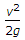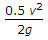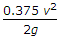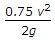# Mechanical Engineering - Hydraulics and Fluid Mechanics

### Exercise :: Hydraulics and Fluid Mechanics - Section 3

6.

The loss of head at entrance in a pipe is (where v = Velocity of liquid in the pipe)

 A.B.C.D.Answer: Option B

Explanation:

No answer description available for this question. Let us discuss.

7.

When an internal mouthpiece is running free, the discharge through the mouthpiece is (where a = Area of mouthpiece, and H = Height of liquid above the mouthpiece)

 A. 0.5 a2gH B. 0.707 a2gH C. 0.855 a2gH D. a2gH

Answer: Option A

Explanation:

No answer description available for this question. Let us discuss.

8.

A flow through an expanding tube at increasing rate is called unsteady non-uniform flow.

 A. Yes B. No

Answer: Option A

Explanation:

No answer description available for this question. Let us discuss.

9.

Water is __________ liquid.

 A. a compressible B. an incompressible

Answer: Option B

Explanation:

No answer description available for this question. Let us discuss.

10.

With an increase in size of tube, the rise or depression of liquid in the tube due to surface tension will

 A. decrease B. increase C. remain unchanged D. depend upon the characteristics of liquid

Answer: Option A

Explanation:

No answer description available for this question. Let us discuss.

#### Current Affairs 2021

Interview Questions and Answers